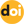The peer-reviewed scientific and technology journal. ISSN: 0204-3327

# A method for statistical comparison of data sets and its uses in analysis of nuclear physics datahttps://doi.org/10.26583/npe.2014.3.05

### UDC: 53.088, 519.23

We propose a method for statistical comparison of two data sets. The method is based on the method of statistical comparison of histograms. Usually a one-dimensional test statistic is used as a measure of distinction of data sets. This test statistic depends on the shape of distributions in data sets. Using the two-dimensional test statistics which is determined via the statistical moments of distribution produced by the calculation of “the significance of deviations” for the corresponding points with observed values is proposed in the paper as a distinction measure between data sets. The significance of deviation in the corresponding points can be considered as a realization of the random variable which is close to a standard normal random variable if we observe the same random value in both data sets. It helps to avoid the dependence of the result on the shape of distributions. The accuracy of the estimator for the measure of distinction is determined by the Monte-Carlo experiment which, by analogy with the construction of repeated samples (resampling) in the bootstrap method, it is possible to call construction of repeated data set (redatasetting). As an estimator of quality of the decision made, it is proposed to use the value which it is possible to call the probability that the decision ‘’data sets are various’’ is correct.

1. EXFOR Library. Available at: http://www-nds.iaea.org/exfor/exfor.htm
2. Barashenkov V.S., Konobeev A.Yu., Korovin Yu.A., Sosnin V.N. Atomnaya Energiya. 1999, no. 87, p. 283.
3. Anrianov A.A., Konobeev A.Yu., Korovin Yu.A., Kuptsov I.S., Stankovskij A.Yu. Izvestiya vuzov. Yadernaya energetika. 2011, no. 2, p. 5.
4. Korovin Yu.A., Maksimushkina A.V. Yadernaya Fizika i Ingeniring. 2014, no. 5, p. 237.
5. Bityukov S.I., Krasnikov N.V., Nikitenko A.N., Smirnova V.V. A method for statistical comparison of histograms. arXiv:1302.2651 - 2013.
6. Bityukov S.I., Krasnikov N.V., Nikitenko A.N., Smirnova V.V. Vestnik RUDN. Seriya: matematika, informatika, fisika. 2014, no.2, p. 324.
7. Bityukov S., Krasnikov N., Nikitenko A., Smirnova V. Eur.Phys.J.Plus. 2013, no.128:143.
8. Porter F. Testing consistency of two histograms. arXiv:0804.0380 - 2008.
9. Efron B. Bootstrap methods: another look at the jackknife. Annals of Statistics. 1979, no. 7, p. 1.
10. Bityukov S.I., Krasnikov N.V. Distinguishability of Hypotheses. Nucl.Inst.&Meth. 2004-A534, p. 152.
11. Korovin Yu.A., Maksimushkina A.V. Izvestiya vuzov. Yadernaya energetika. 2014, no. 2, p. 51.